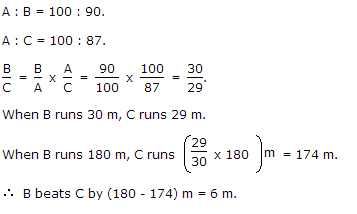3
Q:

# In a 100 m race, A beats B by 10 m and C by 13 m. In a race of 180 m, B will beat C by

 A) 5.4 m B) 4.5 m C) 5 m D) 6 m

Explanation:Q:

In a race of 1000 m, A can beat by 100 m, in a race of 800m, B can beat C by 100m. By how many meters will A beat C in a race of 600 m ?

 A) 127.5 m B) 254 m C) 184 m D) 212 m

Explanation:

When A runs 1000 m, B runs 900 m and when B runs 800 m, C runs 700 m.

When B runs 900 m, distance that C runs = (900 x 700)/800 = 6300/8 = 787.5 m.

In a race of 1000 m, A beats C by (1000 - 787.5) = 212.5 m to C.

In a race of 600 m, the number of meters by which A beats C

= (600 x 212.5)/1000 = 127.5 m.

35 6505
Q:

In a race of 1000 meters, A can beat B by 100 meters, in a race of 800 meters, B can beat C by 100 meters. By how many meters will A beat C in a race of 600 meters?

 A) 125.5 meters B) 126.5 meters C) 127.5 meters D) 128.5 meters

Explanation:

When A runs 1000 meters, B runs 900 meters and when B runs 800 meters, C runs 700 meters.

Therefore, when B runs 900 meters, the distance that C runs = (900 x 700)/800 = 6300/8 = 787.5 meters.

So, in a race of 1000 meters, A beats C by (1000 - 787.5) = 212.5 meters to C.

So, in a race of 600 meters, the number of meters by Which A beats C = (600 x 212.5)/1000 = 127.5 meters.

33 23908
Q:

A can give B 100 meters start and C 200 meters start in a kilometer race. How much start can B give C in a kilometer race?

 A) 110.12 meters B) 111.12 meters C) 112.12 meters D) 113.12 meters

Explanation:

A runs 1000 meters while B runs 900 meters and C runs 800 meters.

Therefore, B runs 900 meters while C runs 800 meters.

So, the number of meters that C runs when B runs 1000 meters = (1000 x 800)/900 = 8000/9 = 888.88 meters

Thus, B can give C (1000 - 888.88) = 111.12 meters start

25 25145
Q:

In a 100 m race, A can beat B by 25 m and B can beat C by 4 m. In the same race, A can beat C by:

 A) 21 m B) 26 m C) 28m D) 29m

Explanation:

A : B = 100 : 75

B : C = 100 : 96.

A : C = (A/B*B/C) = (100/75*100/96) = 100/72  =100:72

A beats C by (100 - 72) m = 28 m.

23 6843
Q:

A runs $123$ times as fast as B. If A gives B a start of 80 m, how far must the winning post be so that A and B might reach it at the same time?

 A) 200 m B) 300m C) 270m D) 160m

Explanation:

Ratio of the speeds of A and B =5 : 1 = 5 :3

Thus, in race of 5 m, A gains 2 m over B.

2 m are gained by A in a race of 5 m.

80 m will be gained by A in race of $52*80$ m =200m

=> Winning post is 200 m away from the starting point.

29 15317
Q:

A can run 22.5 m while B runs 25 m. In a kilometre race B beats A by:

 A) 100 m B) 75 m C) 25 m D) 50m

Explanation:

When B runs 25 m, A runs 45/2 m

When B runs 1000 m, A runs (45/2*1/25*1000)m = 900 m.

B beats A by 100 m.

45 33122
Q:

In a 200 metres race A beats B by 35 m or 7 seconds. A's time over the course is:

 A) 40 sec B) 47sec C) 33sec D) none of these

Explanation:

B runs 35 m in 7 sec.

B covers 200 m in (7/35*200) = 40 sec.

B's time over the course = 40 sec.

A's time over the course (40 - 7) sec = 33 sec.

41 30505
Q:

In a game of 100 points, A can give B 20 points and C 28 points. Then, B can give C:

 A) 8 points B) 10 points C) 14points D) 40points

Explanation:

A : B = 100 : 80.

A : C = 100 : 72.

B:C = B/A*A/C = 80/10*100/72 = 10/9 = 100/90 = 100:90

B can give C 10 points